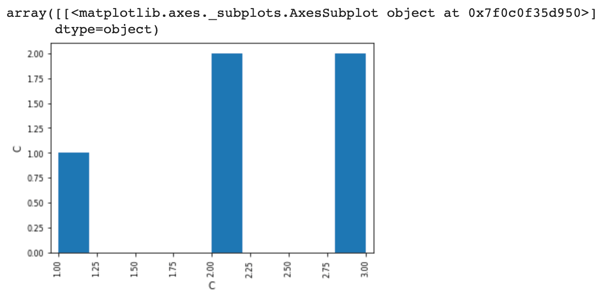Related Tags

python
communitycreator

# What are four underrated pandas functions?

## 1) pandas.pivot_table

• Using this function, we can make a pivot table as a dataframe.

• Format :

pandas.pivot_table(data, values=None, index=None, columns=None, aggfunc='mean', fill_value=None, margins=False, dropna=True, margins_name='All', observed=False)

• Arguments :
1. data : It consists of a dataframe.

2. values :It is the column that we need to aggregate. This is optional to use.

3. index: Enables us to group the values in a row form.

4. columns: Enables us to group the values in a column form.

5. aggfunc: We can pass a list of functions with which we can aggregate the values of the dataframe. If we use the sum function, we can aggregate values by sum.

6. fill_value: We can use this to replace missing values.

7. margins: We use this to add all the rows and columns.

8. dropna: In this, we should not include any columns whose entries are all NaN.

9. margins_name: It contains the name of the row or the column that will contain the total.

10. observed: It is used when the groups are categorical.

import pandas as pd
import numpy as np
df = pd.DataFrame({"A": ["Hey", "Hey", "there", "Hey", "there"],
"B": ["egg", "sandwich", "egg", "sandwich", "egg"],
"C": [1, 2, 2, 3, 3]
})

table = pd.pivot_table(df, values='C', index=['A'],
columns=['B'],fill_value=0)
print(table)

## 2) pandas.DataFrame.describe

• using this function we can find some statistical data about the dataset such as count, mean, frequency, etc.

• The type of statistical data that will show is different for objects and numerical data.

• Format :

pandas.DataFrame.describe(percentiles=None, include=None, exclude=None, datetime_is_numeric=False)

• Arguments :
1. percentiles: A list of numbers that allows us to decide the marks of only the 75th percentile of students.

2. include: With this, we can choose what data types to include.

3. exclude: With this, we can choose what data types we want to exclude.

4. datetime_is_numeric: This will decide whether or not we will treat DateTime data types as a numeric.

import pandas as pd
import numpy as np
df = pd.DataFrame({"A": ["Hey", "Hey", "there", "Hey", "there"],
"B": ["egg", "sandwich", "egg", "sandwich", "egg"],
"C": [1, 2, 2, 3, 3]
})
print(df.describe())
#Using df from above code

## 3) pandas.Series.combine

• We can combine the values of two series using the function below.
• Format :
Series.combine(other, func, fill_value=None)

• Here, we are combining the series in such a manner that we get a series that has max values

• Arguments :

1. other: It is the series value that needs to be combined.

2. func: It takes 2 scalars as an input and returns the elements.

3. fill_value: We can use this to replace missing values.

import pandas as pd
s1 = pd.Series({'MCD': 190.0, 'BK': 240.0})

s2 = pd.Series({'MCD': 278.0, 'BK': 200.0, 'duck': 120.0})

print(s1.combine(s2, max))

### 4) pandas.plotting.scatter_matrix

• It is used to draw a matrix of scatter plots.

• Format :

pandas.plotting.scatter_matrix(frame, alpha=0.5, figsize=None, ax=None, grid=False, diagonal='hist', marker='.', density_kwds=None, hist_kwds=None, range_padding=0.05, **kwargs)

• Arguments :
1. frame: It stands for DataFrame.

2. alpha: With this, you can decide how much transparency you want to apply. The value should usually be a float.

3. figsize: It takes in two inputs from the user both in float. The input should be given in terms of the tuple, which denotes width and height, respectively (width, height).

4. ax: It is a Matplotlib axis object.

5. grid: It takes a boolean value by default. Its value is false, but by setting this to True, it will show you the grid.

6. diagonal:{‘hist’, ‘kde’}: Type ‘kde’ for Kernel Density Estimation and ‘hist’ for Histogram plot.

7. marker: It is Matplotlib marker type.

8. density_kwds: It is passed on to kernel density and used to find the estimated plot.

9. hist_kwds: It is the keyword arguments that are passed on to the hist function.

10. range_padding: It is the relative extension of the axis range. The axis of x and y with respect to (x_max - x_min or y_max - y_min),the default value is given as 0.05.

11. **kwargs: Keyword arguments need to be passed on to the scatter function.

import pandas as pd

df = pd.DataFrame({"A": ["Hey", "Hey", "there", "Hey", "there"],
"B": ["egg", "sandwich", "egg", "sandwich", "egg"],
"C": [1, 2, 2, 3, 3]
})
pd.plotting.scatter_matrix(df, alpha=0.2)RELATED TAGS

python
communitycreator

CONTRIBUTOR